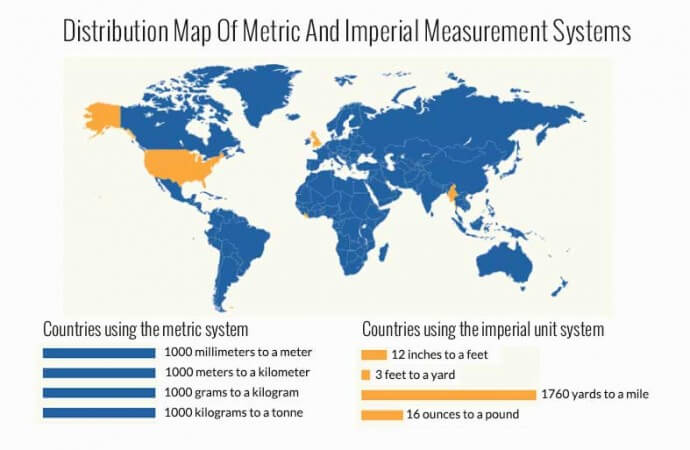• LATEST
• 2015-06-18T07:24:27+00:002015-06-12T04:36:22+00:002015-05-29T04:16:44+00:00
728 x 90

# All you need to know about metric conversion### If you’ve ever been frazzled trying to think about how to convert American or Imperial measurements into metric, you’re not alone. In 1999, some of the brightest people in the world working at NASA managed to crash the Mars Climate Orbiter into the red planet, all because of confusion between traditional units and metric. Well,

If you’ve ever been frazzled trying to think about how to convert American or Imperial measurements into metric, you’re not alone. In 1999, some of the brightest people in the world working at NASA managed to crash the Mars Climate Orbiter into the red planet, all because of confusion between traditional units and metric. Well, worry no more! We’re going to give you all you need to know about converting to metric and back.

## The Metric System

Although a pound is a decent weight for a cake and an inch is about the distance halfway down your thumb, traditional measurement systems don’t necessarily make a lot of sense. For example, why are there 12 inches in every foot, but only 3 feet in every yard? Even more complicated is the fact that different units with the same names are used in different places. Why are there 128 fluid ounces in 1 US gallon, but 160 ounces in a UK gallon, but still 4 quarts in each (US and UK quarts are also different)?

The metric system was designed as a way to do away with all the confusion and to make life easier for those of us who are terrible at doing fractions. In fact, this system was invented in France in 1799 and has since been exported around the world, which also makes communication and international trade much simpler, except in the few countries who haven’t managed to change to the “new” system yet. In metric, whether you’re talking about length, distance, volume, weight, or even temperature, everything divides by 10, 100, or 1000.

### Divide by 10 / 100 / 1000

How many milligrams in a gram? How many milliliters in a liter? How many centimeters in a meter? The answers to all of these questions can be found by learning just a few simple terms.

For numbers less than 1: milli = one thousandth

Ex. One thousandth of a meter is a millimeter. There are 1000 millimeters in a meter.

centi = one hundredth

Ex. One hundredth of a meter is a centimeter. There are 100 centimeters in a meter.

deci = one tenth

Ex. One tenth of a meter is a decimeter. There are 10 decimeters in a meter.

For numbers greater than 1: eca = ten

Ex. One decameter is 10 meters. There are 10 meters in a decameter. (this term is rarely used)

hecto = one hundred

Ex. One hectometer is 100 meters. There are 100 meters in a hectometer. (this term is rarely used)

kilo = one thousand

Ex. One kilometer is 1000 meters. There are 1000 meters in a kilometer.

### Distance

The normal unit in metric for measuring distance is the kilometer (1000 meters). One mile is the same as 1.6 kilometers, which is more than 1.5 times bigger.

If you know miles and you want to find out kilometers:
# of miles X 1.609 = # of kilometers

If you know kilometers and you want to find out miles:
# of kilometers / 1.609 = # of miles

### Length

The normal unit in metric for measuring length is the meter (1000 millimeters or 100 centimeters). One meter is the same as 3.28 feet. One meter is also the same as 39.37 inches.

If you know feet and you want to find out meters:
# of feet X 3.28 = # of meters

If you know meters and you want to find out feet:
# of feet / 3.28 = # of meters

NOTE: If you know length in feet and inches, for example, 6’2”, you will need to convert this into only feet (=6.167 feet) or only inches (=74 inches) before you can convert to metric!

For measuring shorter lengths, especially in carpentry and building, millimeters are used. There are 25.4 millimeters in 1 inch.

If you know inches and you want to find out millimeters:
# of inches X 25.4 = # of millimeters

If you know millimeters and you want to find out inches:
# of millimeters / 25.4 = # of meters

### Volume

The normal unit in metric for measuring volume is the liter (1000 milliliters or 100 centiliters). One liter is the same as 0.0296 ounces. One gallon (US) is also the same as 3.785 liters.

If you know ounces and you want to find out liters:
# of ounces X 0.0296 = # of liters (divide by 1000 for # of milliliters)

If you know liters and you want to find out ounces:
# of liters / 0.0296 = # of ounces

If you know gallons and you want to find out liters:

# of gallons X 3.785 = # of liters
If you know liters and you want to find out gallons:
# of liters / 3.785 = # of gallons

### Weight

The normal unit in metric for measuring weight is the gram (1000 grams = 1 kilogram). One gram is the same as 0.0353 ounces.

If you know ounces and you want to find out liters:
# of ounces / 0.0353 = # of grams (divide by 1000 for # of milliliters)

If you know grams and you want to find out ounces:
# of liters X 0.0353 = # of ounces

One pound is also the same as 0.456 kilograms.

If you know pounds and you want to find out kilograms:
# of pound X 0.456 = # of kilograms

If you know kilograms and you want to find out pounds:
# of kilograms / 0.456 = # of pounds

#### Featured Videos